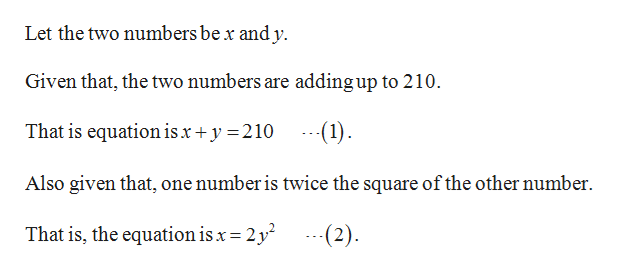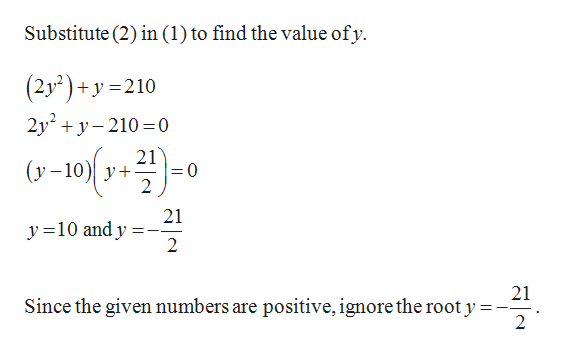# Construct a system of nonlinear equations to describe the given behavior, then solve for the requested solutions. Two positive numbers add up to 210. One number is twice the square of the other number. What are the numbers? (Enter your answers as a comma-separated list.)

Question
2 views

Construct a system of nonlinear equations to describe the given behavior, then solve for the requested solutions.

Two positive numbers add up to 210. One number is twice the square of the other number. What are the numbers? (Enter your answers as a comma-separated list.)

check_circle

Step 1help_outlineImage TranscriptioncloseLet the two numbers be x and y. Given that, the two numbers are adding up to 210. That is equation isx +y 210 -1) Also given that, one number is twice the square of the other number 2 (2) That is, the equation is x 2y fullscreen
Step 2help_outlineImage TranscriptioncloseSubstitute (2) in (1) to find the value ofy (2y2)+y 210 2y2 210 0 21 = 0 2 (y-10y 21 y10 and y 2 21 Since the given numbers are positive, ignore the root y = 2 fullscreen

### Want to see the full answer?

See Solution

#### Want to see this answer and more?

Solutions are written by subject experts who are available 24/7. Questions are typically answered within 1 hour.*

See Solution
*Response times may vary by subject and question.
Tagged in

### Other